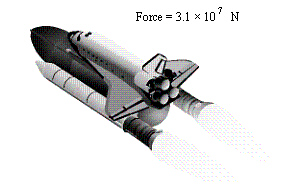Name:    Quiz 10.5-10.7

Multiple Choice
Identify the choice that best completes the statement or answers the question.

Write the number in standard form.

1.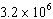a. 320,000 c. 3,200,000 b. 19,200,000 d. 32,000,000

2.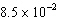a. 0.085 c. 0.17 b. 0.85 d. 0.0085

Write the number in scientific notation.

3.

431,000
 a.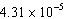c.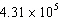b.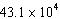d.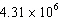4.

0.00006
 a.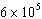c.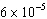b.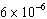d.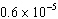5.

Each year, more than 274,000,000 gallons of water are used to make man-made snow at a ski resort. Write this number in scientific notation.
 a.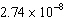gal c.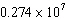gal b.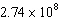gal d.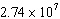gal

6.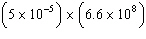a.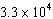c.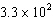b.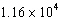d.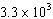7.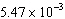+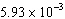a.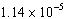c.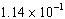b.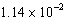d.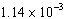8.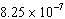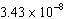a.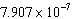c.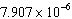b.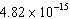d.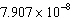9.

(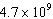) ¸ (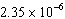)
 a.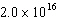c.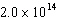b.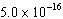d.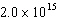Tell whether the number is written in scientific notation. Explain.

1.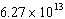2.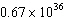3.

The table shows the speed of sound through various substances.
 Substance Gold Glassware Water Air Brass Speed (km/h) 1.2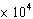25.4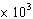1.21.7a.   Through which substance does sound travel fastest?
b.   Through which substance does sound travel slowest?

4.

The table shows the equatorial circumference of three planets in our solar system. Order the circumferences from least to greatest.

 Planet Circumference (km) Uranus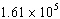Neptune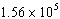Mercury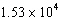5.

The power of a space shuttle is the combined force of the thrusters multiplied by the velocity.
The velocity of the shuttle is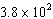meters per second. What is the power (in newton-meters per second) of the shuttle?